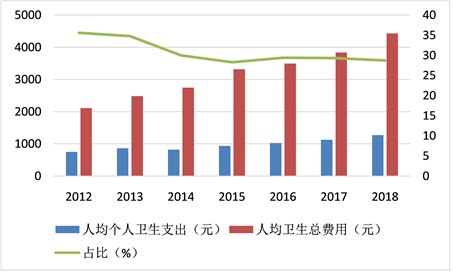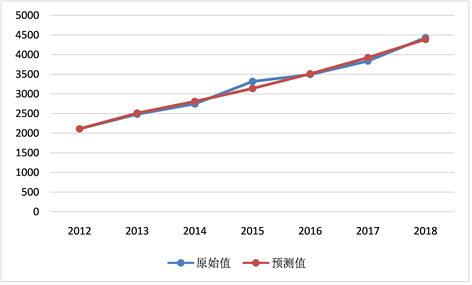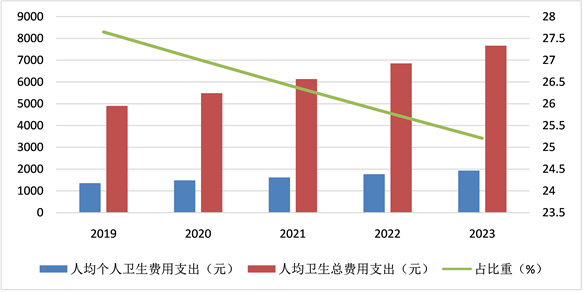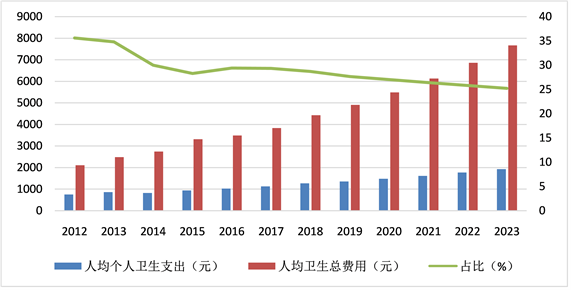﻿ 重庆市城乡居民个人医疗负担特点及预测研究

# 重庆市城乡居民个人医疗负担特点及预测研究Characteristics and Prediction of Personal Medical Burden of Urban and Rural Residents in Chongqing

Abstract: By calculating the personal health expenditure of urban and rural residents in Chongqing, this paper studies the situation and characteristics of personal expenditure of urban and rural residents in Chongqing in recent 7 years, and carries out future prediction research according to its characteristics, in order to further optimize the fairness of health financing structure.

1. 资料来源与方法

1.1. 资料来源

1.2. 研究方法

1.3. 统计学方法

2. 结果

2.1. 基于GM(1,1)模型的个人卫生支出占卫生总费用比例预测Table 1. Personal health expenditure accounts for the proportion of total health expensesFigure 1. Proportion of per capita health expenditure of urban and rural residents in Chongqing from 2012 to 2018

2.2. 级比检验，建模可行性分析

${X}^{\left(0\right)}=\left({X}^{\left(0\right)}\left(1\right),{X}^{\left(0\right)}\left(2\right),\cdots ,{X}^{\left(0\right)}\left(7\right)\right)=\left(751.34,863.95,823.07,937.38,1026.7,1125.13,1271.6\right)$ ；其次，求级比值； $\sigma =\left(0.8697,1.0497,0.8781,0.9130,0.9125,0.8848\right)$，发现所有的级比值都恰好在区间(0.778800783, 1.284025417)内，因此模型的建立能进行。

2.3. 灰色GM(1,1)模型的建立

${X}^{\left(1\right)}=\left({X}^{\left(1\right)}\left(1\right),{X}^{\left(1\right)}\left(2\right),\cdots ,{X}^{\left(1\right)}\left(7\right)\right)=\left(751.34,1615.29,2438.36,3375.74,4402.44,5527.57,6799.17\right)$。其次，构造均值数列，即

${Z}^{\left(1\right)}=\left({Z}^{\left(1\right)}\left(2\right),\cdots ,{Z}^{\left(1\right)}\left(7\right)\right)=\left(1183.315,2026.825,2907.05,3889.09,4965.005,6163.37\right)$

$Z=\left(\begin{array}{c}\frac{1}{2}\left[{X}^{\left(1\right)}\left(1\right)+{X}^{\left(1\right)}\left(2\right)\right]\\ \frac{1}{2}\left[{X}^{\left(1\right)}\left(2\right)+{X}^{\left(1\right)}\left(3\right)\right]\\ \frac{1}{2}\left[{X}^{\left(1\right)}\left(3\right)+{X}^{\left(1\right)}\left(4\right)\right]\\ \frac{1}{2}\left[{X}^{\left(1\right)}\left(4\right)+{X}^{\left(1\right)}\left(5\right)\right]\\ \frac{1}{2}\left[{X}^{\left(1\right)}\left(5\right)+{X}^{\left(1\right)}\left(6\right)\right]\\ \frac{1}{2}\left[{X}^{\left(1\right)}\left(6\right)+{X}^{\left(1\right)}\left(7\right)\right]\end{array}\right)=\left(\begin{array}{c}1183.315\\ 2026.825\\ 2907.05\\ 3889.09\\ 4965.005\\ 6163.37\end{array}\right)$

$Y=\left(\begin{array}{c}{X}^{\left(0\right)}\left(2\right)\\ {X}^{\left(0\right)}\left(3\right)\\ {X}^{\left(0\right)}\left(4\right)\\ {X}^{\left(0\right)}\left(5\right)\\ {X}^{\left(0\right)}\left(6\right)\\ {X}^{\left(0\right)}\left(7\right)\end{array}\right)=\left(\begin{array}{c}863.95\\ 823.07\\ 937.38\\ 1026.7\\ 1125.13\\ 1271.6\end{array}\right)$

$B=\left(\begin{array}{c}-{Z}^{\left(1\right)}\left(2\right)\\ -{Z}^{\left(1\right)}\left(3\right)\\ -{Z}^{\left(1\right)}\left(4\right)\\ -{Z}^{\left(1\right)}\left(5\right)\\ -{Z}^{\left(1\right)}\left(6\right)\\ -{Z}^{\left(1\right)}\left(7\right)\end{array}\right)=\left(\begin{array}{cc}-1183.315& 1\\ -2026.825& 1\\ -2907.05& 1\\ -3889.09& 1\\ -4965.005& 1\\ -6163.37& 1\end{array}\right)$

${\Lambda }_{P}={\left({\Lambda }_{a},{\Lambda }_{b}\right)}^{\text{T}}={\left({B}^{\text{T}}B\right)}^{-1}{B}^{\text{T}}Y=\left(\begin{array}{c}-0.08850\\ 696.24540\end{array}\right)$，由此可得： $a=-0.08850$$b=696.24540$$\frac{b}{a}=-7867.429515$${X}^{\left(0\right)}\left(1\right)-\frac{b}{a}=8618.769515$。最后，解得重庆市城乡人均个人卫生费用支出的预测模型为： ${\Lambda }_{{X}^{\left(1\right)}\left(k+1\right)}=8618.769515{\text{e}}^{0.08850k}-7867.429515$，通过累减还原得到预测的值。

${X}^{\left(0\right)}\left(1\right)-\frac{b}{a}=21270.88652$。因此，最终可以得到其GM(1,1)模型为：

${\Lambda }_{{X}^{\left(1\right)}\left(k+1\right)}=21270.88652{\text{e}}^{0.11159k}-19160.38652$，通过累减还原得到预测的值。

2.4. 模型的拟合检验

GM(1,1)模型需要精度合适才能用以对未来的预测。通常，为了检验预测模型是不是可靠，常用后验差比值法。

2.5. 对2019年~2023年重庆市人均个人卫生支出进行预测Table 2. Model accuracy level evaluation standardFigure 2. Per capita personal health expenditure fitting effectFigure 3. Effectiveness of total per capital health expensesTable 3. Forecast value of per capita personal health expenditure and its proportion in total health expenditure from 2019 to 2023Figure 4. Per capita personal health expenditure and accounting map of Chongqing Province 2019~2023Figure 5. Per capita personal health expenditure and its proportion in Chongqing from 2012 to 2023

3. 讨论与结论

3.1以总额预付为主的混合支付模式有助于促进医疗保险制度的发展

3.2完善医疗保障体系，发挥商业健康险的作用

 丁海峰, 高凯, 姜茂敏. 基于灰色GM(1,1)模型的上海市卫生总费用预测研究[J]. 医学与社会, 2020, 33(6): 42-46.

 耿新丽, 张云霞. 重庆市卫生总费用的结构分析[J]. 财经界(学术版), 2018, 23(25): 40-41.

 洪媛媛. 广东省卫生总费用影响因素主成分回归分析[J]. 卫生软科学, 2015, 29(12): 756-759.

Top